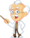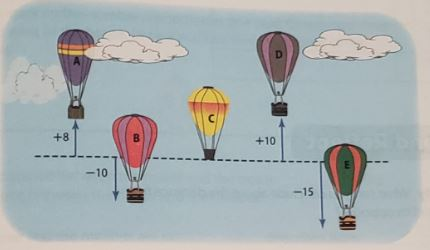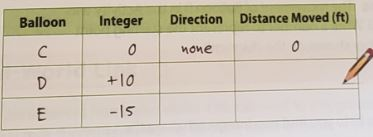Homework Explained - Math Practice 101Dear guest, you are not a registered member. As a guest, you only have read-only access to our books, tests and other practice materials.

As a registered member you can:

 Question 1 How can a number line help you find two integers that are the same distance from zero? Type below:(show solution) Question 2 Several hot air baloons were flying at the same height. The dashed line below represents their starting point. Which two baloons moved the same distance but in oposite directions? Type below:(show solution) Question 3 In the diagram below, +8 means Balloon A climbed 8 feet and -10 means Balloon A moved down 10 feet.Use the diagram to compare the distance each balloon moved. Question 3 Step 1 Complete the chart to compare the distance each balloon moved from the dashed line.Type below:(show solution) Question 3 Step 2 Determine which two balloons moved the same distance away from the dashed line. So, balloon __ and balloon __ moved __  feet from the dashed line, Type below:(show solution)